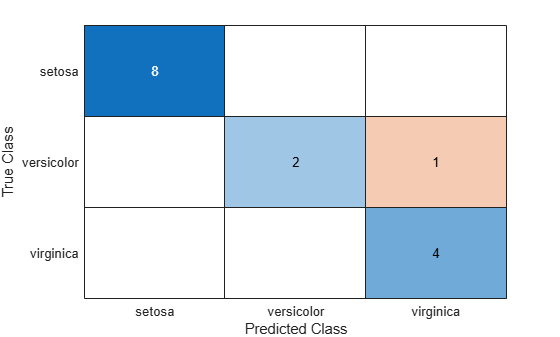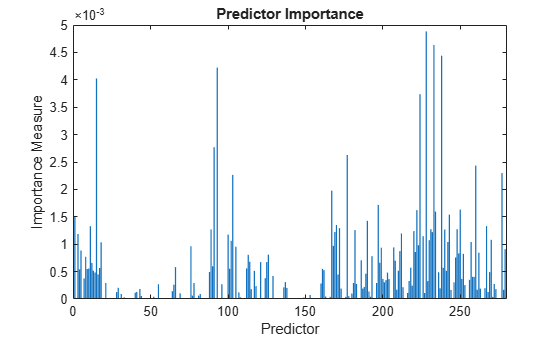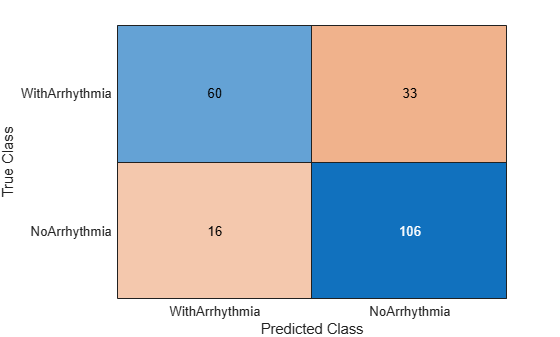# predict

Classify observations using ensemble of classification models

## Syntax

``labels = predict(ens,X)``
``labels = predict(ens,X,Name=Value)``
``````[labels,scores] = predict(___)``````

## Description

example

````labels = predict(ens,X)` returns a vector of predicted class labels for the predictor data in the table or matrix `X`, based on the trained classification ensemble model (full or compact) `ens`. ```
````labels = predict(ens,X,Name=Value)` specifies additional options using one or more name-value arguments. For example, you can specify the weak learners to use for predictions, and perform computations in parallel. ```
``````[labels,scores] = predict(___)``` also returns a matrix of classification scores indicating the likelihood that a label comes from a particular class, using any of the input argument combinations in the previous syntaxes. For each observation in `X`, the predicted class label corresponds to the maximum score among all classes.```

## Input Arguments

expand all

Full classification ensemble model, specified as a `ClassificationEnsemble` model object trained with `fitcensemble`, or a `CompactClassificationEnsemble` model object created with `compact`.

Predictor data to be classified, specified as a numeric matrix or a table.

Each row of `X` corresponds to one observation, and each column corresponds to one variable.

For a numeric matrix:

• The variables that make up the columns of `X` must have the same order as the predictor variables used to train `ens`.

• If you trained `ens` using a table (for example, `tbl`), `X` can be a numeric matrix if `tbl` contains only numeric predictor variables. To treat numeric predictors in `tbl` as categorical during training, specify categorical predictors using the `CategoricalPredictors` name-value argument of `fitcensemble`. If `tbl` contains heterogeneous predictor variables (for example, numeric and categorical data types) and `X` is a numeric matrix, `predict` issues an error.

For a table:

• `predict` does not support multicolumn variables or cell arrays other than cell arrays of character vectors.

• If you trained `ens` using a table (for example, `tbl`), all predictor variables in `X` must have the same variable names and data types as those used to train `ens` (stored in `ens.PredictorNames`). However, the column order of `X` does not need to correspond to the column order of `tbl`. `tbl` and `X` can contain additional variables, such as response variables and observation weights, but `predict` ignores them.

• If you trained `ens` using a numeric matrix, then the predictor names in `ens.PredictorNames` must be the same as the corresponding predictor variable names in `X`. To specify predictor names during training, use the `PredictorNames` name-value argument of `fitcensemble`. All predictor variables in `X` must be numeric vectors. `X` can contain additional variables, such as response variables and observation weights, but `predict` ignores them.

### Name-Value Arguments

Specify optional pairs of arguments as `Name1=Value1,...,NameN=ValueN`, where `Name` is the argument name and `Value` is the corresponding value. Name-value arguments must appear after other arguments, but the order of the pairs does not matter.

Before R2021a, use commas to separate each name and value, and enclose `Name` in quotes.

Example: `predict(ens,X,Learners=[1 2 3 5],UseParallel=true)` specifies to use the first, second, third, and fifth learners in the ensemble `ens`, and to perform computations in parallel.

Indices of weak learners in the ensemble to use in `predict`, specified as a vector of positive integers in the range [1:`ens.NumTrained`]. By default, all learners are used.

Example: `Learners=[1 2 4]`

Data Types: `single` | `double`

Option to use observations for learners, specified as a logical matrix of size `N`-by-`T`, where:

When `UseObsForLearner(i,j)` is `true` (default), learner `j` is used in predicting the class of row `i` of `X`.

Example: `UseObsForLearner=logical([1 1; 0 1; 1 0])`

Data Types: `logical matrix`

Flag to run in parallel, specified as a numeric or logical `1` (`true`) or `0` (`false`). If you specify `UseParallel=true`, the `predict` function executes `for`-loop iterations by using `parfor`. The loop runs in parallel when you have Parallel Computing Toolbox™.

Example: `UseParallel=true`

Data Types: `logical`

## Output Arguments

expand all

Predicted class labels, returned as a categorical, character, logical, or numeric array, or a cell array of character vectors. `labels` has the same data type as the labels used to train `ens`. (The software treats string arrays as cell arrays of character vectors.)

The `predict` function classifies an observation into the class yielding the highest score. For an observation with `NaN` scores, the function classifies the observation into the majority class, which makes up the largest proportion of the training labels.

Class scores, returned as a numeric matrix with one row per observation and one column per class. For each observation and each class, the score represents the confidence that the observation originates from that class. A higher score indicates a higher confidence. For more information, see Score (ensemble).

## Examples

expand all

Load Fisher's iris data set. Determine the sample size.

```load fisheriris N = size(meas,1);```

Partition the data into training and test sets. Hold out 10% of the data for testing.

```rng(1); % For reproducibility cvp = cvpartition(N,'Holdout',0.1); idxTrn = training(cvp); % Training set indices idxTest = test(cvp); % Test set indices```

Store the training data in a table.

```tblTrn = array2table(meas(idxTrn,:)); tblTrn.Y = species(idxTrn);```

Train a classification ensemble using AdaBoostM2 and the training set. Specify tree stumps as the weak learners.

```t = templateTree('MaxNumSplits',1); Mdl = fitcensemble(tblTrn,'Y','Method','AdaBoostM2','Learners',t);```

Predict labels for the test set. You trained model using a table of data, but you can predict labels using a matrix.

`labels = predict(Mdl,meas(idxTest,:));`

Construct a confusion matrix for the test set.

`confusionchart(species(idxTest),labels)``Mdl` misclassifies one versicolor iris as virginica in the test set.

Create an ensemble of boosted trees and inspect the importance of each predictor. Using test data, assess the classification accuracy of the ensemble.

Load the arrhythmia data set. Determine the class representations in the data.

```load arrhythmia Y = categorical(Y); tabulate(Y)```
``` Value Count Percent 1 245 54.20% 2 44 9.73% 3 15 3.32% 4 15 3.32% 5 13 2.88% 6 25 5.53% 7 3 0.66% 8 2 0.44% 9 9 1.99% 10 50 11.06% 14 4 0.88% 15 5 1.11% 16 22 4.87% ```

The data set contains 16 classes, but not all classes are represented (for example, class 13). Most observations are classified as not having arrhythmia (class 1). The data set is highly discrete with imbalanced classes.

Combine all observations with arrhythmia (classes 2 through 15) into one class. Remove those observations with an unknown arrhythmia status (class 16) from the data set.

```idx = (Y ~= "16"); Y = Y(idx); X = X(idx,:); Y(Y ~= "1") = "WithArrhythmia"; Y(Y == "1") = "NoArrhythmia"; Y = removecats(Y);```

Create a partition that evenly splits the data into training and test sets.

```rng("default") % For reproducibility cvp = cvpartition(Y,"Holdout",0.5); idxTrain = training(cvp); idxTest = test(cvp);```

`cvp` is a cross-validation partition object that specifies the training and test sets.

Train an ensemble of 100 boosted classification trees using `AdaBoostM1`. Specify to use tree stumps as the weak learners. Also, because the data set contains missing values, specify to use surrogate splits.

```t = templateTree("MaxNumSplits",1,"Surrogate","on"); numTrees = 100; mdl = fitcensemble(X(idxTrain,:),Y(idxTrain),"Method","AdaBoostM1", ... "NumLearningCycles",numTrees,"Learners",t);```

`mdl` is a trained `ClassificationEnsemble` model.

Inspect the importance measure for each predictor.

```predImportance = predictorImportance(mdl); bar(predImportance) title("Predictor Importance") xlabel("Predictor") ylabel("Importance Measure")```Identify the top ten predictors in terms of their importance.

```[~,idxSort] = sort(predImportance,"descend"); idx10 = idxSort(1:10)```
```idx10 = 1×10 228 233 238 93 15 224 91 177 260 277 ```

Classify the test set observations. View the results using a confusion matrix. Blue values indicate correct classifications, and red values indicate misclassified observations.

```predictedValues = predict(mdl,X(idxTest,:)); confusionchart(Y(idxTest),predictedValues)```Compute the accuracy of the model on the test data.

```error = loss(mdl,X(idxTest,:),Y(idxTest), ... "LossFun","classiferror"); accuracy = 1 - error```
```accuracy = 0.7731 ```

`accuracy` estimates the fraction of correctly classified observations.

expand all

## Alternative Functionality

To integrate the prediction of an ensemble into Simulink®, you can use the ClassificationEnsemble Predict block in the Statistics and Machine Learning Toolbox™ library or a MATLAB® Function block with the `predict` function. For examples, see Predict Class Labels Using ClassificationEnsemble Predict Block and Predict Class Labels Using MATLAB Function Block.

When deciding which approach to use, consider the following:

• If you use the Statistics and Machine Learning Toolbox library block, you can use the Fixed-Point Tool (Fixed-Point Designer) to convert a floating-point model to fixed point.

• Support for variable-size arrays must be enabled for a MATLAB Function block with the `predict` function.

• If you use a MATLAB Function block, you can use MATLAB functions for preprocessing or post-processing before or after predictions in the same MATLAB Function block.

## Version History

Introduced in R2011a